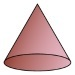##### Notes

Daily Math Practice provides a week's worth of problems ideal for morning work or review. Week 18 reviews standards from previous weeks and supports Common Core Mathematics Standards K.OA.A.1, K.OA.A.2, & K.OA.A.3.

##### Print Instructions

NOTE: Only your test content will print.
To preview this test, click on the File menu and select Print Preview.

See our guide on How To Change Browser Print Settings to customize headers and footers before printing.

# Kindergarten - Daily Math Practice - Week 18 (Kindergarten)

Print Test (Only the test content will print)
 DAILY MATH PRACTICE - WEEK 18 DAY 1 DAY 2 Add the dots.1 + 2 = Add the dots.2 + 3 = Cole baked 2 apples pies for the fair. He also baked 2 pumpkin pies. How many pies did Cole bake? Draw a picture:Answer:pies There are 3 bean plants growing in the garden. Abby plants 1 more bean plant. How many bean plants are in the garden now?Write a number sentence:Answer:bean plants Circle the addition sentence for the model.3 + 4 = 70 + 7 = 7 Circle the addition sentence for the model.1 + 6 = 72 + 5 = 7 Draw an X on the solid shape.Count forward. Write the next number.8, 9, 10,

 DAILY MATH PRACTICE - WEEK 18 DAY 3 DAY 4 Add the dots.1 + 4 = Add the dots.3 + 1 = Mia finds 3 shells on the beach. She then finds 1 more shell. How many shells does Mia find?Draw a picture:Answer:shells There are 2 boys in the dance class. There are also 3 girls. How many children are in the dance class?Write a number sentence:Answer:children Circle the addition sentence for the model.1 + 6 = 72 + 5 = 7 Circle the addition sentence for the model.4 + 3 = 75 + 2 = 7 Circle letter in front of M.R M Q Write the missing number.17 = 1 ten +onesYou need to be a HelpTeaching.com member to access free printables.